## In the Real World

Remember all that stuff about left-hand sums and right-hand sums? That's why your calculator works. Your calculator doesn't know what sin(3) is any better than you do, but it's able to take accurate approximations quickly.

We talked about how if you take enough rectangles, you can guarantee that the approximation is within a certain amount of the real answer. A calculator screen only displays ten or so decimal places, so the calculator needs to guarantee that its approximation is correct for as many decimal places as the screen will show.

It's cool to think that you can say your approximation is no more than a certain distance from the real answer even though you don't know what the real answer is.

### General Riemann Sums

A general Riemann sum is what we get when we estimate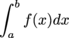using rectangles and not necessarily finding the rectangle on each sub-interval the same way.

For the sake of the pictures, we'll use a non-negative f. We split up the interval [a, b] into any n sub-intervals we like, not necessarily of the same size: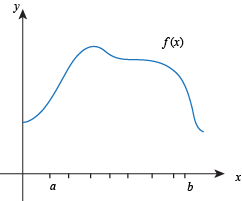On each sub-interval, we pick a special x. It might be the left endpoint of the sub-interval, or the right-endpoint, or the midpoint, or somewhere else, and we don't need to pick the special x the same way on each interval. For each sub-interval, we use the function value at the special x as the height of the rectangle: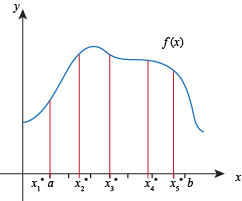To write this in symbols instead of pictures, we use the counter i to keep track of which sub-interval we're on. The counter i will run from 1 to n, since there are n sub-intervals. Sub-interval i has width Δxi and special x value x*i. This means the rectangle on sub-interval i has width Δxi and height f (x*i):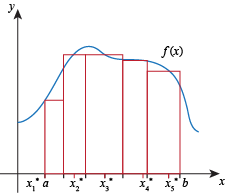Adding up the areas of all the rectangles, our general Riemann sum estimates the area between f and the x axis to be

f (x*1x1 + f (x*2x2 + ... + f (x*ixi + ... + f (x*{n – 1}xn – 1 + f (x*nxn.

We can write this more tidily with summation notation as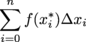• ### How to Solve a Math Problem

• Figure out what the problem is asking.
• Solve the problem.

### Sample Problem

Find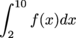given that f is odd and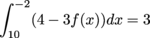• Figure out what the problem is asking.

We need to use various properties of integrals to rearrange this equation until there's ain it somewhere. Then we solve for• Solve the problem.

There are so many ways to start this, deciding which to do is a project in itself. We'll do one way to get the answer here, and we'll do it a different way to check our work.

First, split up the integral to get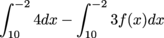Work with the first term first. Since the first term is the integral of the constant 4 on an interval of length 12, that term is equal to 48.

Now we have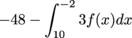Switch the limits and the sign: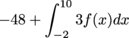Pull out the constant: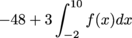Since f is odd,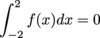, so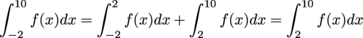Now we have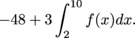We know this is supposed to be equal to 3: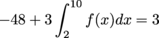Solving,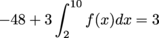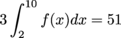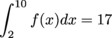.

If we do the problem again, but a different way, we should get the same answer.

Starting with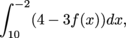switch the limits and the sign first this time: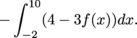Now we'll split up the integral, but we need to be careful that the negative sign still affects everything: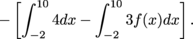Since f is odd, 3f(x) is also odd. This means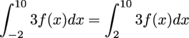, since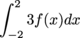is zero. Put this back into our expression: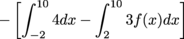.

Now we pull out the constant: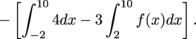We can substitute 48 for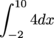like we did before: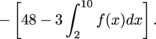It's time to start solving. We know this is all supposed to equal 3.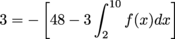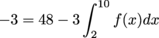Adding 3 and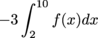to both sides,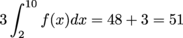and again we get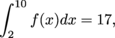which is reassuring.

## This is a premium product